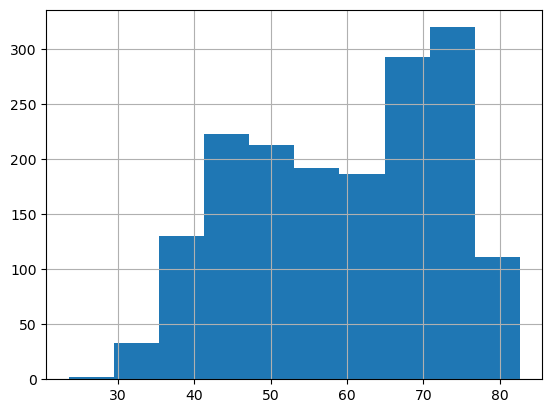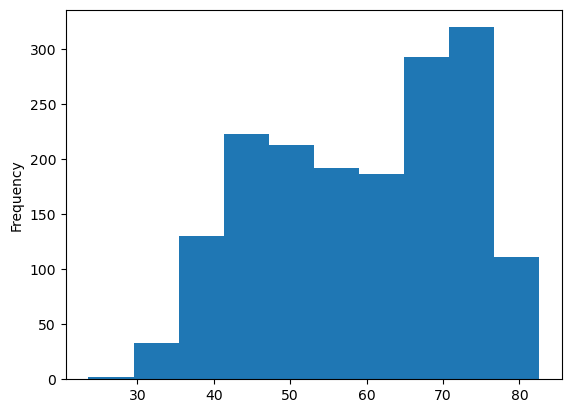## Libraries

Pandas is a popular open-source Python library used for data manipulation and analysis. It provides data structures and functions that make working with structured data, such as tabular data (like `Excel` spreadsheets or `SQL` tables), easy and intuitive.

To install Pandas, you can use the following command in your command-line interface (such as `Terminal` or `Command Prompt`):

`pip install pandas`

Matplotlib functionalities have been integrated into the pandas library, facilitating their use with `dataframes` and `series`. For this reason, you might also need to import the matplotlib library when building charts with Pandas.

This also means that they use the same functions, and if you already know Matplotlib, you'll have no trouble learning plots with Pandas.

``````import pandas as pd
import matplotlib.pyplot as plt``````

## Dataset

In order to create graphics with Pandas, we need to use pandas objects: `Dataframes` and `Series`. A dataframe can be seen as an `Excel` table, and a series as a `column` in that table. This means that we must systematically convert our data into a format used by pandas.

Since histograms need quantitative variables, we will get the Gap Minder dataset using the `read_csv()` function. The data can be accessed using the url below.

``````url = 'https://raw.githubusercontent.com/holtzy/The-Python-Graph-Gallery/master/static/data/gapminderData.csv'

## Histogram with the hist() function

Once we've opened our dataset, we'll now create the graph. The following displays the distribution of the life expectancy using the `hist()` function. This is probably one of the shortest ways to display a histogram in Python.

``````df["lifeExp"].hist()
plt.show()``````## Histogram with the plot() function

We'll now look at how to create a histogram using the `plot()` function. This function is very general and therefore requires more arguments to be specified when it is called.

The main argument is `kind`. This specifies the type of chart we want (in our case it's `'hist'`). For example, we could have put `'line'` for a line chart but not `'scatter'` since we need 2 variables for a scatter plot (this will trigger an error).

``````df["lifeExp"].plot(kind='hist')
plt.show()``````## Histogram with the plot.hist() function

And now we'll look at how to create a histogram using the `plot.hist()` function. This function is a combination of the previous 2, but is no more complicated.

``````df["lifeExp"].plot.hist()
plt.show()``````## Going further

This post explains how to create a simple histogram with pandas in 3 different ways.

For more examples of how to create or customize your plots with Pandas, see the pandas section. You may also be interested in how to customize your histograms with Matplotlib and Seaborn.

## Contact & Edit

👋 This document is a work by Yan Holtz. You can contribute on github, send me a feedback on twitter or subscribe to the newsletter to know when new examples are published! 🔥